Gurchetan1000 Singh — Published On January 15, 2018 and Last Modified On May 12th, 2023

## Introduction

In this tutorial, I’ll introduce you to anova, its objectives, statistical tests, test examples, statistical analysis, and the different ANOVA techniques used for making the best decisions. We’ll take a few cases and try to understand the techniques for getting the results. We will also be leveraging the use of Excel to understand these concepts.Source: Megapixl

You must know the basics of anova statistics to understand this topic. Knowledge of t-tests and Hypothesis testing would be an additional benefit. And we believe the best way to learn statistics is by doing. That’s how we follow in the Introduction to Data Science course, where we provide a comprehensive introduction to descriptive and inferential statistics.

Learning Objectives

• In this tutorial, we will learn about Anova and its different types.
• You will familiarize yourself with the different terminologies associated with Anova.
• You will also learn how to calculate Anova In Microsoft Excel.

## What Is Analysis of Variance (ANOVA)?

Buying a new product or testing a new technique but not sure how it stacks up against the alternatives? It’s an all too familiar situation for most of us. Most options sound similar to each other, so picking the best out of the lot is a challenge.

Consider a scenario where we have three medical treatments for patients with similar diseases. Once we have the test results, one approach is to assume that the treatment which took the least time to cure the patients is the best among them. What if some of these patients had already been partially cured, or if any other medication was already working on them?

In order to make a confident and reliable decision, we will need evidence to support our approach. This is where the concept of ANOVA comes into play.

A common approach to figuring out a reliable treatment method would be to analyze the days the patients took to be cured. We can use a statistical technique to compare these three treatment samples and depict how different these samples are from one another. Such a technique, which compares the samples based on their means, is called ANOVA.

Analysis of variance (ANOVA) is a statistical technique used to check if the means of two or more groups are significantly different from each other. ANOVA checks the impact of one or more factors by comparing the means of different samples. We can use ANOVA to prove/disprove whether all the medication treatments were equally effective.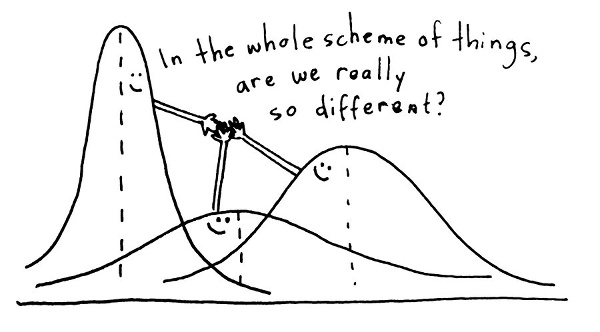Source: Questionpro

Another measure to compare the samples is called a t-test. When we have only two samples, t-test, and ANOVA give the same results. However, using a t-test would not be reliable in cases with more than 2 samples. If we conduct multiple t-tests for comparing more than two samples, it will have a compounded effect on the error rate of the result.

Before we start with the ANOVA applications, I would like to introduce some common terminologies used in the technique.

### Grand Mean

Mean is a simple or arithmetic average of a range of values. There are two kinds of means that we use in ANOVA calculations, which are separate sample means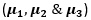and the grand mean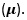. The grand mean is the mean of sample means or the mean of all observations combined, irrespective of the sample.

### Hypothesis

Considering our above medication example, we can assume that there are 2 possible cases – either the medication will have an effect on the patients or it won’t. These statements are called Hypothesis. A hypothesis is an educated guess about something in the world around us. It should be testable either by experiment or observation.

Just like any other kind of hypothesis that you might have studied in statistics, ANOVA also uses a Null hypothesis and an Alternate hypothesis. The Null hypothesis in ANOVA is valid when all the sample means are equal, or they don’t have any significant difference. Thus, they can be considered as a part of a larger set of the population. On the other hand, the alternate hypothesis is valid when at least one of the sample means is different from the rest of the sample means. In mathematical form, they can be represented as: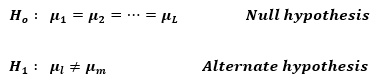Where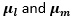belonging to any two sample means out of all the samples considered for the test. In other words, the null hypothesis states that all the sample means are equal or the factor did not have any significant effect on the results. Whereas, the alternate hypothesis states that at least one of the sample means is different from another. But we still can’t tell which one specifically. For that, we will use other methods that we will discuss later in this article.

### Between Group Variability

Consider the distributions of the below two samples. As these samples overlap, their individual means won’t differ by a great margin. Hence the difference between their individual and grand means won’t be significant enough.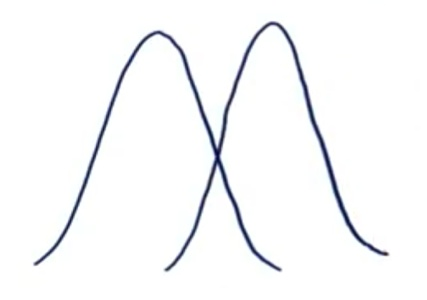Now consider these two sample distributions. As the samples differ from each other by a big margin, their individual means would also differ. The difference between the individual means and grand mean would, therefore, also be significant.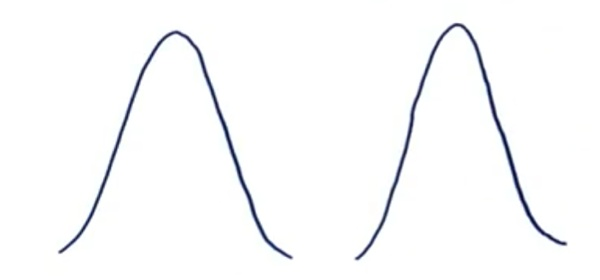Such variability between the distributions is called Between-group variability. It refers to variations between the distributions of individual groups (or levels) as the values within each group differ.
Each sample is examined, and the difference between its mean and grand mean is calculated to calculate the variability. If the distributions overlap or are close, the grand mean will be similar to the individual means, whereas if the distributions are far apart, the difference between means and grand mean would be large.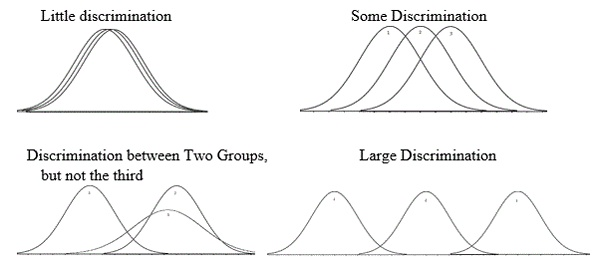Source: Psychstat – Missouri State

We will calculate Between Group Variability just as we calculate the standard deviation. Given the sample means and Grand mean, we can calculate it as follows: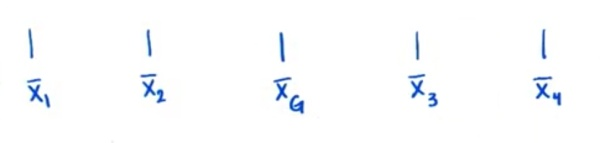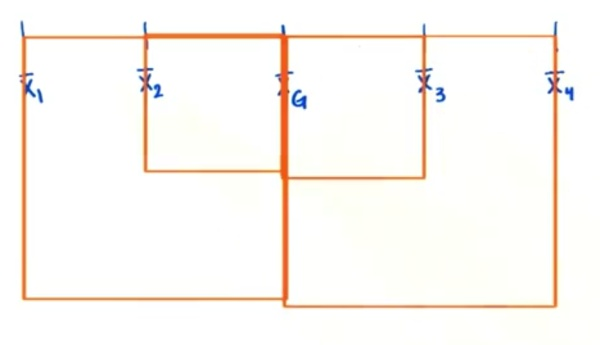Source: Udacity

We also want to weigh each squared deviation by the sample size. In other words, a deviation is given greater weight if it’s from a larger sample. Hence, we’ll multiply each squared deviation by each sample size and add them. This is called the sum-of-squares for between-group variability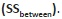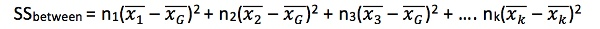We must do one more thing to derive a good measure of between-group variability. Again, recall how we calculate the sample standard deviation.

We find the sum of each squared deviation and divide it by the degrees of freedom. For our between-group variability, we will find each squared deviation, weigh them by their sample size, sum them up, and divide by the degrees of freedom (), which in the case of between-group variability is the number of sample means (k) minus 1.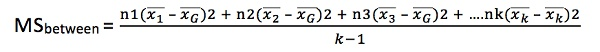### Within Group Variability

Consider the given distributions of three samples. As the spread (variability) of each sample increases, their distributions overlap, and they become part of a big population.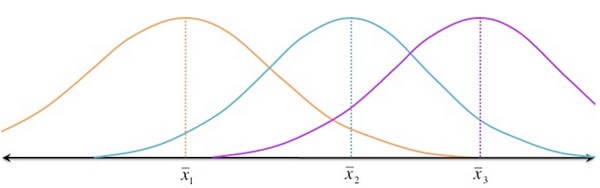Now consider another distribution of the same three samples but with less variability. Although the means of samples are similar to those in the above image, they seem to belong to different populations.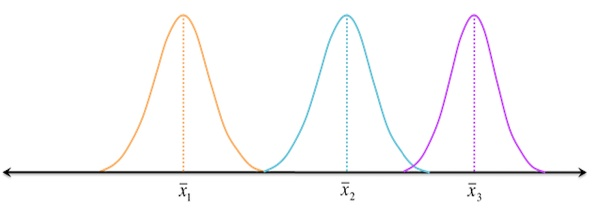Source: TurntheWheelsandBox

Such variations within a sample are denoted by Within-group variation. It refers to variations caused by differences within individual groups (or levels), as not all the values within each group are the same. Each sample is looked at on its own, and variability between the individual points in the sample is calculated. In other words, no interactions between samples are considered.

We can measure Within-group variability by looking at how much each value in each sample differs from its respective sample mean. So first, we’ll take the squared deviation of each value from its respective sample mean and add them up. This is the sum of squares for within-group variability.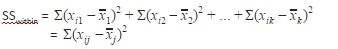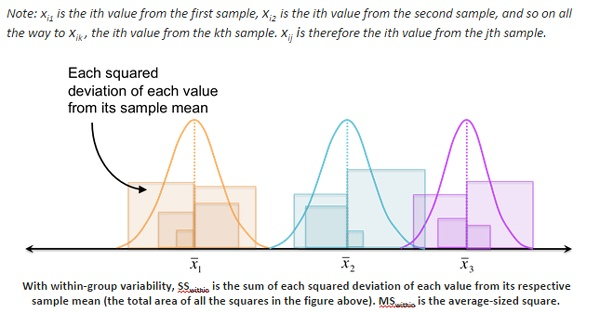Source: TurntheWheelsandBox

Like between-group variability, we then divide the sum of squared deviations by the degrees of freedom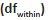to find a less-biased estimator for the average squared deviation (essentially, the average-sized square from the figure above). Again, this quotient is the mean square, but for within-group variability: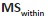. This time, the degrees of freedom is the sum of the sample sizes (N) minus the number of samples (k). Another way to look at degrees of freedom is that have the total number of values (N) and subtract 1 for each sample: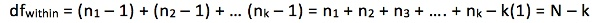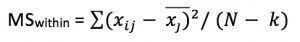### F-Statistic (F-test)

The statistic that measures whether the means of different samples are significantly different is called the F-Ratio. The lower the F-Ratio, the more similar will the sample means be. In that case, we cannot reject the null hypothesis.

F = Between-group variability / Within-group variability

This above formula is pretty intuitive. The numerator term in the F-statistic calculation defines the between-group variability. As we read earlier, the sample means to grow further apart as between-group variability increases. In other words, the samples are likelier to belong to different populations.
This F-statistic calculated here is compared with the F-critical value for concluding. In terms of our medication example, if the value of the calculated F-statistic is more than the F-critical value (for a specific α/significance level), then we reject the null hypothesis and can say that the treatment had a significant effect.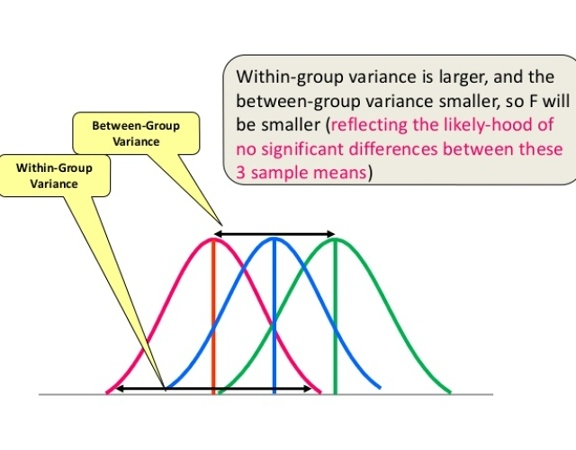Source: Dr. Asim’s Anatomy Cafe

Unlike the z and t-distributions, the F-distribution has no negative values because between and within-group variability are always positive due to squaring each deviation.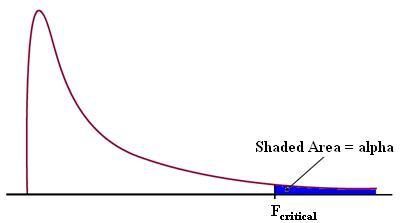Source: Statistics How To

Therefore, there is only one critical region in the right tail (shown as the blue-shaded region above). If the F-statistic lands in the critical region, we can conclude that the means are significantly different, and we reject the null hypothesis. Again, we must find the critical value to determine the cut-off for the critical region. We’ll use the F-table for this purpose.

We need to look at different F-values for each alpha/significance level because the F-critical value is a function of two things: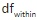and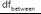.

## One Way ANOVA or Single Factor Anova

As we now understand the basic terminologies behind ANOVA, let’s dive deep into its implementation using a few examples.

A recent study claims that music in a class enhances the concentration and consequently helps students absorb more information. As a teacher, your first reaction would be skepticism.

What if it affected the results of the students in a negative way? Or what kind of music would be a good choice for this? Considering all this, it would be immensely helpful to have some proof that it actually works.

To figure this out, we implemented it on a smaller group of randomly selected students from three different classes. The idea is similar to conducting a survey. We took three different groups of ten randomly selected students (all of the same age) from three different classrooms. Each classroom was provided with a different environment for students to study. Classroom A had constant music being played in the background, classroom B had variable music being played, and classroom C was a regular class with no music playing. After one month, we conducted a test for all the three groups and collected their test scores. The test scores that we obtained were as follows: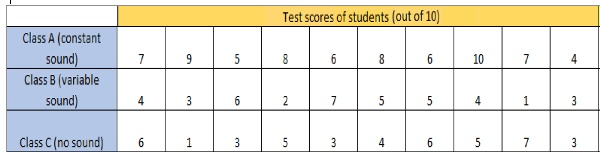Now, we will calculate the means and the Grand mean.
So, in our case,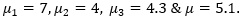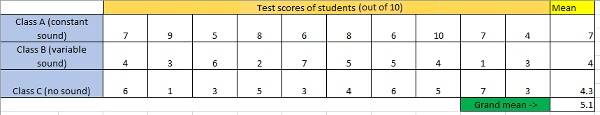Looking at the above table, we might assume that the mean score of students from Group A is definitely greater than the other two groups, so the treatment must be helpful. Maybe it’s true, but there is also a slight chance that we happened to select the best students from class A, which resulted in better test scores (remember, the selection was made at random). This leads to a few questions, like:

1. How do we decide that these three groups performed differently because of the different situations and not merely by chance?
2. In a statistical sense, how different are these three samples from each other?
3. What is the probability of group A students performing differently than the other two groups?

To answer all these questions, first, we will calculate the F-statistic, which can be expressed as the ratio Between Group variability and Within Group Variability.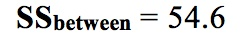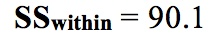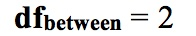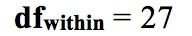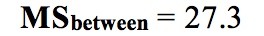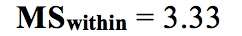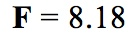Let’s complete the ANOVA test for our example with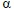= 0.05.

### Limitations of One-Way ANOVA

A one-way ANOVA tells us that at least two groups are different from each other. But it won’t tell us which groups are different. If our test returns a significant f-statistic, we may need to run a post-hoc test to tell us exactly which groups differ in means. Below I have mentioned the steps to perform one-way ANOVA in Microsoft Excel along with a post-hoc test.

### Step-by-Step to Perform One-Way ANOVA With Post-hoc Test in Excel 2013

Step 1: Input your data into columns or rows in Excel. For example, if three groups of students for music treatment are being tested, spread the data into three columns.
Step 2: Click the “Data” tab and then click “Data Analysis.” If you don’t see Data Analysis, load the ‘Data Analysis Toolpak’ add-in.
Step 3: Click “ANOVA Single Factor” and then click “OK.”
Step 4: Type an input range into the Input Range box. For example, if the data is in cells A1 to C10, type “A1:C10” into the box. Check the “Labels in the first row” if we have column headers, and select the Rows radio button if the data is in rows.
Step 5: Select an output range. For example, click the “New Worksheet” radio button.
Step 6: Choose an alpha level. For most hypothesis tests, 0.05 is standard.
Step 7: Click “OK.” The results from ANOVA will appear in the worksheet.
The results for our example look like this:

Here, we can see that the F-value is greater than the F-critical value for the alpha level selected (0.05). Therefore, we have evidence to reject the null hypothesis and say that at least one of the three samples have significantly different means and thus belongs to an entirely different population.
Another measure for ANOVA is the p-value. If the p-value is less than the alpha level selected (which it is, in our case), we reject the Null Hypothesis.

There are various methods for finding out which samples represent two different populations. I’ll list some for you:

We won’t be covering all of these here in this article, but I suggest you go through them.

Now to check which samples had different means, we will take the Bonferroni approach and perform the post hoc test in Excel through following steps:
Step 8: Again, click on “Data Analysis” in the “Data” tab and select “t-Test: Two-Sample Assuming Equal Variances,” and click “OK.”
Step 9: Input the range of Class A column in Variable 1 Range box and range of Class B column in Variable 2 Range box. Check the “Labels” if you have column headers in the first row.
Step 10: Select an output range. For example, click the “New Worksheet” radio button.
Step 11: Perform the same steps (Step 8 to step 10) for Columns of Class B – Class C and Class A – Class C.

The results will look like this: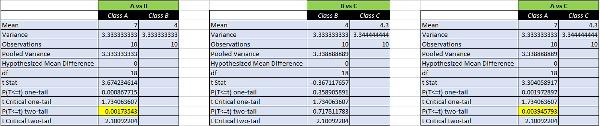Here, we can see that the p-value of (A vs B) and (A vs C) is less than the alpha level selected (alpha = 0.05). This means that groups A and B & groups A and C have less than a 5% chance of belonging to the same population. Whereas for (B vs C), it is much greater than the significance level. This means that B and C belong to the same population. So, it is clear that A (constant music group) belongs to an entirely different population. Or we can say that the constant music had a significant effect on students’ performance.

Voila! The music experiment actually helped in improving the results of the students.

Another effect size measure for one-way ANOVA is called Eta squared. It works in the same way as R2 for t-tests. It is used to calculate how much proportion of the variability between the samples is due to the between-group difference. It is calculated as: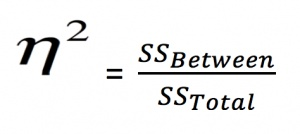For the above example: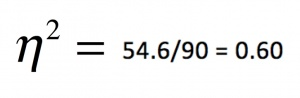Hence 60% of the difference between the scores is because of the approach that was used. Rest 40% is unknown. Hence, the Eta square helps us conclude whether the independent variable really impacts the dependent variable or whether the difference is due to chance or any other factor.

There are commonly two types of ANOVA tests for univariate analysis – One-Way ANOVA and Two-Way ANOVA. One-way ANOVA is used when we are interested in studying the effect of one independent variable (IDV)/factor on a population. In contrast, Two-way ANOVA is used for studying the effects of two factors on a population simultaneously. For multivariate analysis, such a technique is called MANOVA or Multi-variate ANOVA.

## Two-Way ANOVA

Using one-way ANOVA, we found out that the music treatment helped improve the test results of our students. But this treatment was conducted on students of the same age. What if the treatment was to affect different age groups of students in different ways? Or maybe the treatment had varying effects depending upon the teacher who taught the class.

Moreover, how can we be sure which factor(s) is affecting the students’ results more? Maybe the age group is a more dominant factor responsible for a student’s performance than the music treatment.

For such cases, when the outcome or dependent variable (in our case, the test scores) is affected by two independent variables/factors, we use a slightly modified technique called two-way ANOVA.
In the one-way ANOVA test, we found that the group subjected to ‘variable music’ and ‘no music at all’ performed more or less equally. It means that the variable music treatment did not have any significant effect on the students.

So, while performing two-way ANOVA, we will not consider the “variable music” treatment for simplicity of calculation. Rather a new factor, age, will be introduced to find out how the treatment performs when applied to students of different age groups. This time our dataset looks like this:

Here, there are two factors – class and age groups with two and three levels, respectively. So we now have six different groups of students based on different permutations of class groups and age groups, and each different group has a sample size of 5 students.

1. Is music treatment the main factor affecting performance? In other words, do groups subjected to different music differ significantly in their test performance?
2. Is age the main factor affecting performance? In other words, do students of different ages differ significantly in their test performance?
3. Is there a significant interaction between the factors? In other words, how do age and music interact with regard to a student’s test performance? For example, it might be that younger and older students reacted differently to such a music treatment.
4. Can any differences in one factor be found within another factor? In other words, can any differences in music and test performance be found in different age groups?

Two-way ANOVA tells us about the main effect and the interaction effect. The main effect is similar to a one-way ANOVA where the effect of music and age would be measured separately. In comparison, the interaction effect is the one where both music and age are considered at the same time.

That’s why a two-way ANOVA can have up to three hypotheses, which are as follows:
Two null hypotheses will be tested if we have placed only one observation in each cell. For this example, those hypotheses will be:
H1: All the music treatment groups have an equal mean score.
H2: All the age groups have an equal mean score.

For multiple cell observations, we would also test a third hypothesis:
H3: The factors are independent, or the interaction effect does not exist.

An F-statistic is computed for each hypothesis we are testing.
Before proceeding with the calculation, look at the image below. It will help us better understand the terms used in the formulas.

The table shown above is known as a contingency table. Here,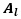it represents the total of the samples based only on factor 1 and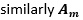represents the total of samples based only on factor 2. We will see in some time that these two are responsible for the main effect produced. Also, a term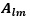is introduced representing the subtotal of factor 1 and factor 2. This term will be responsible for the interaction effect produced when both the factors are considered simultaneously. And we are already familiar with the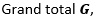, which is the sum of all the observations (test scores), irrespective of the factors.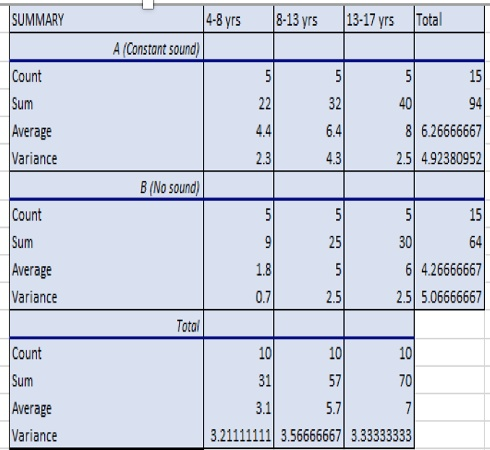We have calculated all the means – sound class mean, age group mean, and mean of every group combination in the above table.

Now, calculate the sum of squares (SS) and degrees of freedom (df) for sound class, age group, and interaction between factor and levels.

We already know how to calculate SS (within)/df (within) in our one-way ANOVA section, but in two-way ANOVA, the formula is different. Let’s look at the calculation of two-way ANOVA: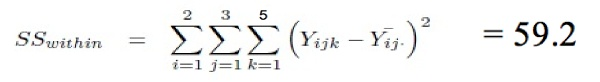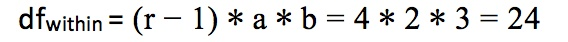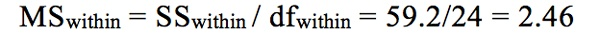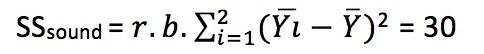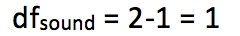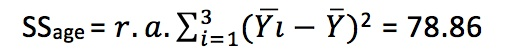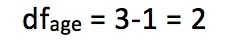In two-way ANOVA, we also calculate SSinteraction and dfinteraction, which defines the combined effect of the two factors.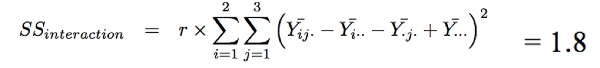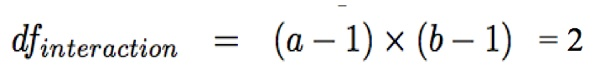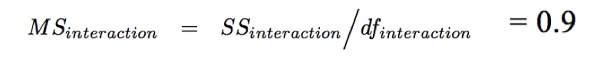Since we have more than one source of variation (main effects and interaction effects), it is obvious that we will have more than one F-statistic also.

Using these variances, we compute the value of F-statistic for the main and interaction effect. So, the values of f-statistic are,
F1 = 12.16
F2 = 15.98
F12 = 0.36
We can see the critical values from the table
Fcrit1 = 4.25
Fcrit2 = 3.40
Fcrit12 = 3.40
Suppose for a particular effect, its F value is greater than its respective F-critical value (calculated using the F-Table). In that case, we reject the null hypothesis for that particular effect.

### Steps to Perform Two-Way ANOVA in Excel 2013

Step 1: Click the “Data” tab and then click “Data Analysis.” If you don’t see the Data analysis option, install the Data Analysis Toolpak.
Step 2: Click “ANOVA two factor with replication” and then click “OK.” The two-way ANOVA window will open.
Step 3: Type an Input Range into the Input Range box. For example, if your data is in cells A1 to A25, type “A1:A25” into the Input Range box. Ensure you include all of your data, including headers and group names.
Step 4: Type a number in the “Rows per sample” box. Rows per sample is actually a bit misleading. What this is asking you is how many individuals are in each group. For example, if you have 5 individuals in each age group, you would type “5” into the Rows per Sample box.
Step 5: Select an Output Range. For example, click the “new worksheet” radio button to display the data in a new worksheet.
Step 6: Select an alpha level. In most cases, an alpha level of 0.05 (5 percent) works for most tests.
Step 7: Click “OK” to run the two-way ANOVA. The data will be returned in your specified output range.
Step 8: Read the results. To figure out if you are going to reject the null hypothesis or not, you’ll basically be looking at two factors:

1. If the F-value (F)is larger than the f critical value (F crit)
2. If the p-value is smaller than your chosen alpha level.

And you are done!
Note: We don’t only have to have two variables to run a two-way ANOVA in Excel 2013. We can also use the same function for three, four, five, or more variables.

The results for two-way ANOVA test on our example look like this:

As you can see in the highlighted cells in the image above, the F-value for sample and column, i.e., factor 1 (music) and factor 2 (age), respectively, are higher than their F-critical values. This means that the factors significantly affect the students’ results, and thus we can reject the null hypothesis for the factors.
Also, the F-value for interaction effect is quite less than its F-critical value, so we can conclude that music and age did not have any combined effect on the population.

## Multi-Variate ANOVA (MANOVA)

Until now, we have been making conclusions about the performance of students based on just one test. Could there be a possibility that the music treatment helped improve the results of a subject like mathematics but would affect the results adversely for a theoretical subject like history?

How can we ensure the treatment won’t be biased in such a case? So again, we take two groups of randomly selected students from a class and subject each group to one kind of music environment, i.e., constant music and no music. But now we thought of conducting two tests (maths and history), instead of just one. This way, we can be sure how the treatment would work for different subjects.

We can say that one IDV/factor (music) will be affecting two dependent variables (maths scores and history scores) now. This kind of problem comes under a multivariate case; the technique we will use to solve it is known as MANOVA. Here, we will work on a specific case called one-factor MANOVA. Let us now see how our data looks:

####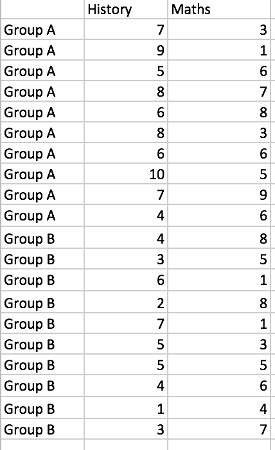Here we have one factor, music, with 2 levels. This factor will affect our two dependent variables, i.e., the test scores in maths and history. Denoting this information in terms of variables, we can say that we have L = 2 (2 different music treatment groups) and P = 2 (maths and history scores).
A MANOVA test also takes into consideration a null hypothesis and an alternate hypothesis.:### Steps to Perform MANOVA in Excel 2013

Step 2: Press “control+m” to open RealStats window
Step 3: Select “Analysis of variance”
Step 4: Select “MANOVA: single factor”
Step 5: Type an Input Range into the Input Range box. For example, if your data is in cells A1 to A25, type “A1:A25” into the Input Range box. Make sure you include all of your data, including headers and group names.
Step 6: Select “Significance analysis”, “Group Means” and “Multiple Anova”.
Step 7: Select an Output Range.
Step 8: Select an alpha level. In most cases, an alpha level of 0.05 (5 percent) works for most tests.
Step 9: Click “OK” to run. The data will be returned in your specified output range.
Step 10: Read the results. To figure out if you are going to reject the null hypothesis or not, you’ll basically be looking at two factors:

1. If the F-value (F)is larger than the f critical value (F crit)
2. If the p-value is smaller than your chosen alpha level.

And you are done!

RealStats add-on shows us the results by different methods. Each one of them denotes the same p-value. We will reject the null hypothesis because the p-value is less than the alpha value. Or in simpler terms, it means that the music treatment did have a significant effect on students’ test results.

But we still cannot tell which subject was affected by the treatment and which was not. This is one of the limitations of MANOVA; even if it tells us whether the effect of a factor on a population was significant, it does not tell us which dependent variable was actually affected by the factor introduced.

For this purpose, we will see the “Multiple ANOVA” table to generate a helpful summary. The result will look like this:

Here, we can see that the P value for history lies in a significant region (since P value is less than 0.025) while for maths, it does not. This means that the music treatment had a significant effect in improving the performance of students in history but did not have any significant effect in improving their performance in maths.

Based on this, we might consider picking and choosing subjects where this music approach can be used.

## Conclusion

I hope this article was helpful and now you’d be comfortable solving similar problems using Analysis of Variance. I suggest you take different kinds of problem statements and take your time to solve them using the above-mentioned techniques.

You should also check out the below two resources to give your data science journey a huge boost:

Key Takeaways

• ANOVA is a statistical formula used to compare variances across the means (or average) of different groups.
• There are two types of commonly used ANOVA; one-way ANOVA and two-way ANOVA.
• To analyze variance (ANOVA), statisticians or analysts use the f-test to compute the feasibility of variability amongst two groups more than the variations observed within the said groups under study.

Q1. What is ANOVA used for in Excel?

A. In Excel, ANOVA is a built-in statistical test used to analyze the variances. For instance, we usually compare the available alternatives when buying a new item, which eventually helps us choose the best from all the available options.

Q2. What is ANOVA? Explain its uses and applications.

A. One can use ANOVA to test for statistical differences between two or more groups to check if there is any significant difference between the means of those groups.

Q3. What is the name of the Excel function for ANOVA?

A. ANOVA is not a function in Excel. In Microsoft Excel, ANOVA is part of Excel’s “Data Analysis” tool.###### Gurchetan1000 Singh

Budding Data Scientist from MAIT who loves implementing data analytical and statistical machine learning models in Python. I also understand big data technology like Hadoop and Alteryx.

### Our Top Authors

•## 18 thoughts on "ANOVA: Complete guide to Statistical Analysis & Applications (Updated 2023)"###### K. Kalyanaraman says:January 15, 2018 at 11:26 am
The alternative hypothesis may be better stated as "at least a pair of means is different" Reply###### Aletta Dieben says:January 15, 2018 at 1:14 pm
Great and pleasant explanation of Anova. Thanks! Reply###### Thomas Richardson says:January 15, 2018 at 5:49 pm
I found the explanation of the 1 way anova great, but couldn't follow the 2 way anova at all. Also is this for repeated measures anova or between participants? I hear they are very different mathematically. Reply###### Gurchetan Singh says:January 16, 2018 at 12:20 pm
Hey Thomas The basic intuition behind One way Anova is to determine the effects of Music treatment on the scores of the students. Similarly in Two way Anova, we determine the effects of 2 variables (Age, Music) on the score of the students. In addition we also check for combined effect of these two variables. In regards to your other query, this is not repeated measures Anova. Repeated measures Anova involves determining the effect of independent variables on the same sample ie determining the effect of music on the same sample of students. This could be done by determining the scores of students without music and comparing it with scores of same students with music treatment. Reply###### JOSE LUIS CRUZ says:January 17, 2018 at 11:46 am
This is what I was waiting for, I hope you have the chance to publish more articles related with statistical tools in Tableau. Best regards Reply###### Gurchetan Singh says:January 17, 2018 at 11:59 am
Thank you Aletta. Happy Learning :) Reply###### Gurchetan Singh says:January 17, 2018 at 2:14 pm
Thank you Jose. There are a few articles on Tableau on the website which you can try. Happy Learning :) Reply###### Arooj says:January 24, 2018 at 3:25 pm
Great article. Thanks for posting. Reply###### chandru says:January 29, 2018 at 12:25 pm###### Murtala says:January 31, 2018 at 8:15 pm
A great piece. I learnt a lot in such a fast and fun way. Thanks a lot! Reply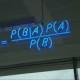###### Jarad says:February 02, 2018 at 2:42 am
You just dropped a knowledge-bomb on me! Thank you Mr. Singh. This was much easier to read than most dense articles around this subject. I will no-doubt be revisiting this again and again for reference. Reply###### Nadir says:February 20, 2018 at 8:04 pm
phew! I am more confident with Anova now!!! Reply###### Luis says:April 06, 2018 at 1:23 am
Great article! Thank you very much for publishing this. I have a question on how it could help me with the following analysis. Any feedback an/or tips would certainly be appreciated. Thank you! I have 5 variables that impact a result, let's call it "TC". When I evaluate each variable independently (with the rest of the variables constant), per plotting these I know they are linear and follow these y=mx+b equations. y1 = -0.9890 * X1 + 8.655, y2 = 0.2340 * X2 + 8.670, y3 = 5.0633 * X3 + 5.037, y4 = -3.3136 * X4 + 12.674, and, y5 = -3.0745 * X5 + 11.285 when, (x1 x2 x3 x4 x5) = (x1, 0, 5, 6.5, 6.5), (0, x2, 5, 6.5, 6.5) (0, 0, x3, 6.5, 6.5), (0, 0, 5, x4, 6.5), and, (0, 0, 5, 6.5, x5) for each of the equations respectively. I also know that x1 is between -5 and 5, x2 is between -5 and 5, and, x3 is between 1 and 7. So, how can I develop a 5-variable equation to be able to change any of these and get a new TC (or y) value as the output? Again, Thank you! Reply###### Gurchetan Singh says:April 06, 2018 at 1:34 pm
Hey I am still not very clear with the question you asked. Try reframing it. Also, try using the Discussion portal for such questions so that the community can answer you better. Reply###### Johnson says:June 28, 2018 at 12:49 am